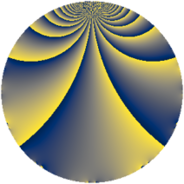# Properties

 Label 3549.2.eLevel $3549$ Weight $2$ Character orbit 3549.e Rep. character $\chi_{3549}(3212,\cdot)$ Character field $\Q$ Dimension $392$ Sturm bound $970$

# Related objects

## Defining parameters

 Level: $$N$$ $$=$$ $$3549 = 3 \cdot 7 \cdot 13^{2}$$ Weight: $$k$$ $$=$$ $$2$$ Character orbit: $$[\chi]$$ $$=$$ 3549.e (of order $$2$$ and degree $$1$$) Character conductor: $$\operatorname{cond}(\chi)$$ $$=$$ $$21$$ Character field: $$\Q$$ Sturm bound: $$970$$

## Dimensions

The following table gives the dimensions of various subspaces of $$M_{2}(3549, [\chi])$$.

Total New Old
Modular forms 512 436 76
Cusp forms 456 392 64
Eisenstein series 56 44 12

## Trace form

 $$392q - 368q^{4} - 4q^{7} + 8q^{9} + O(q^{10})$$ $$392q - 368q^{4} - 4q^{7} + 8q^{9} + 12q^{15} + 336q^{16} + 20q^{18} + 4q^{21} + 16q^{22} + 328q^{25} + 28q^{28} + 20q^{30} - 44q^{36} - 24q^{37} + 4q^{42} - 32q^{43} + 24q^{46} + 20q^{49} + 20q^{51} - 32q^{57} - 24q^{58} + 28q^{60} - 8q^{63} - 304q^{64} + 32q^{67} + 8q^{70} - 64q^{72} - 24q^{79} - 48q^{81} + 48q^{84} + 16q^{85} - 64q^{88} + 52q^{93} - 20q^{99} + O(q^{100})$$

## Decomposition of $$S_{2}^{\mathrm{new}}(3549, [\chi])$$ into newform subspaces

The newforms in this space have not yet been added to the LMFDB.

## Decomposition of $$S_{2}^{\mathrm{old}}(3549, [\chi])$$ into lower level spaces

$$S_{2}^{\mathrm{old}}(3549, [\chi]) \cong$$ $$S_{2}^{\mathrm{new}}(273, [\chi])$$$$^{\oplus 2}$$